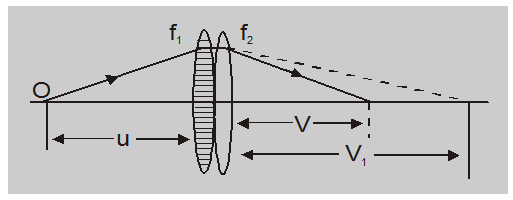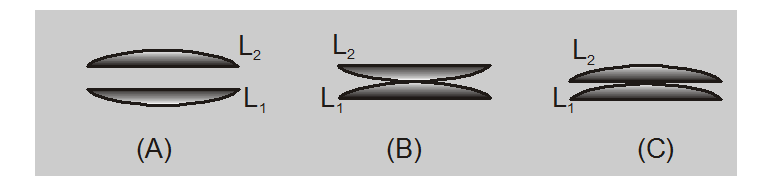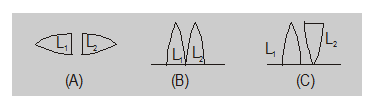Most Affordable JEE | NEET | 8,9,10 Preparation by Kota's Top IITian Doctor Faculties

# Combination of lenses and mirrors - Ray Optics - eSaralHey, do you want to learn about the Combination of lenses and mirrors? If yes. Then you are at the right place.

## Combination of Lenses and Mirror:

When several lenses or mirrors are used co-axially, the image formation is considered one after another in steps. The image formed by the lens facing the object serves as an object for the next lens or mirror the image formed by the second lens (or mirror) acts as an object for the third and so on. The total magnification is such situations will be given by

$\mathrm{m}=\frac{\mathrm{I}}{\mathrm{o}}=\frac{\mathrm{I}_{1}}{\mathrm{o}} \times \frac{\mathrm{I}_{2}}{1_{1}} \times \ldots$

i.e.$\quad m=m_{1} \times m_{2} \times \ldots \ldots$

In the case of two thin lenses in contact if the first lens of focal length $\mathrm{f}_{1}$ forms the image $\left.\right|_{1}$ (of an object)at a distance $\mathrm{V}_{1}$ from it.

$\frac{1}{v_{1}}-\frac{1}{u}=\frac{1}{f_{1}}$ ……(1)now the image $\left.\right|_{1}$ will act as an object for the second lenses and if the second lenses form an image. I at a distance v from it

$\frac{1}{v}-\frac{1}{v_{1}}=\frac{1}{f_{2}}$......(2)

So adding Eqn. (1) and (2) we have

$\frac{1}{v}-\frac{1}{u}=\frac{1}{f_{1}}+\frac{1}{f_{2}}$

or $\frac{1}{v}-\frac{1}{u}=\frac{1}{f}$

with $\quad \frac{1}{f}=\frac{1}{f_{1}}+\frac{1}{f_{2}}$

i.e. the combination behaves as a single lens of equivalent focal length f given by –

$\frac{1}{F}=\frac{1}{f_{1}}+\frac{1}{f_{2}}$

or $P=P_{1}+P_{2}$.....(3)

note : If the two thin lenses are separated by a distance d apart F is given by

$\frac{1}{F}=\frac{1}{f_{1}}+\frac{1}{f_{2}}-\frac{d}{f_{1} f_{2}}$

or $P=P_{1}+P_{2}-P_{1} P_{2} d$

here is it worthy to note that -

Special points:

1. If two thin lenses of equal focal length, but of opposite nature (i.e. one convergent and other divergent) are put in contact, the resultant focal length of the combination be

$\frac{1}{\mathrm{~F}}=\frac{1}{+\mathrm{f}}+\frac{1}{-\mathrm{f}}=0$

i.e.

$F=\infty$ and $P=0$ i.e. the system will behave like a plane. glass plate.

2. If two thin lens of the same nature are put in contact then as

$\frac{1}{\mathrm{~F}}=\frac{1}{\mathrm{f}_{1}}+\frac{1}{\mathrm{f}_{2}}$

$\frac{1}{F}>\frac{1}{f_{1}}$ and $\frac{1}{\mathrm{~F}}>\frac{1}{\mathrm{f}_{2}}$

i.e. $\quad F i.e. the resultant focal length will be lesser than the smallest individual. 3. If two thin lenses of opposite nature with different focal lengths are put in contact the resultant focal length will be of the same nature as that of the lens of shorter focal length but its magnitude will be more than that of shorter focal length. 4. If a lens of focal length f is divided into two equal parts as in figure (A) each part has a focal length f' then as$\frac{1}{f}=\frac{1}{f^{\prime}}+\frac{1}{f^{\prime}}f^{\prime}=2 f$i.e. each part have focal length 2f now if these parts are put in contact as in (B) or (C) the resultant focal length of the combination will be$\frac{1}{F}=\frac{1}{2 f}+\frac{1}{2 f}$i.e.$F=f($=initial value$)$5. If a lens of focal length f is cut in two equal parts as shows in each will have focal length f. Now of these parts are put in contact as shown in the resultant length will be$\frac{1}{\mathrm{~F}}=\frac{1}{\mathrm{f}}+\frac{1}{\mathrm{f}}$i.e.$\mathrm{F}=(\mathrm{f} / 2)$However if the two parts are put in contact as shown in first will behave as the convergent lens of focal length f while the other divergent of same focal length (being thinner near the axis) so in this situation.$\frac{1}{F}=\frac{1}{+f}+\frac{1}{-f}$i.e.$-F=\infty$or$P=0\$

So, that's all from this article. I hope you get the idea about the Combination of lenses and mirrors. If you found this article informative and valuable then please share it with your friends. If you have any confusion related to this topic then you can ask in the comments section down below.

For a better understanding of this chapter, please check the detailed notes of Physics, Ray Optics. To watch Free Learning Videos on physics by Saransh Gupta sir Install the eSaral App.